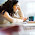## Sunday, November 25, 2012

### Introducing Genifer, 2012

I started working on AGI in 2004, and my greatest "achievement" up to 2012 (8 years) is the invention of a new logic.  All my work thus far is focused on the thinking aspect of AGI, deferring the aspects of planning and acting.

## Concept composition logic

Consider a complex sentence:
"John walks to church slowly, thinking about Turing"

It can be decomposed into:

1. "John walks to church"
2. "John walks slowly"
3. "John walks thinking about Turing"
Using the Genifer GUI (github code is here) we can manually create a graph that represent the sentence structure as follows:

The GUI automatically generates a "factorization" formula of the graph.

The key idea of the graph is that if a word A modifies word B, we create an arrow $A \multimap B$.  More than 1 word can modify a target, but each word can only modify 1 target.  This ensures that the result is a tree, and can be expressed as a sum of products, using $+$ and $\times$.

We can use the distributive law of multiplication to expand the factorized formula.

A problematic case:  If we translate these 2 sentences into logic:

1. "John gives the ball to Mary"  and
2. "John gives $2 to Kevin" After factorizing and expanding we get: 1. john gives to mary 2. john gives the ball 3. john gives to kevin 4. john gives$2
so it may be confused that John gives $2 to Mary. But I reckon that the distributive law is not the real cause of the error. The error arises because the 2 "johns" are the same John but the 2 "gives" are different instances of giving. ## Variable-free logic This is an example of the logical definition of "downstairs" from the SUMO ontology: On the left side is the logic formula, on the right the English translation. As you can see from the translation, it uses a lot of "$n^{th}$objects" which are logic variables. But notice that all these variables invariably belong to some classes (such as "building_level"). So we can just do without variables and directly use ontology classes for matching. For example: "All girls like to dress pretty" "Jane is a girl" implies "Jane likes to dress pretty". In logic the steps would be: girls like to dress pretty jane$\in$girls$\Rightarrow$jane likes to dress pretty because the "girl" atom in both formulas match, so "jane" is substituted into the conclusion. ## Parsing of natural language This is an example parse tree of an English sentence: A simple grammar rule is: "A noun phrase (NP) followed by a verb phrase (VP) is a sentence (S)". In classical AI, which uses first-order predicate logic, we have to parse the English sentence to obtain the parse tree (syntax parsing), and then translate the parse tree into logic (semantic parsing). The final logic formula would be like: loves(john, mary). The semantic parsing process is complicated and involves using$\mathbf{\lambda}$-calculus to represent sentence fragments. In our new logic we can just write: NP$\circ$VP$\in$S And we have these as data: loves$\circ$mary$\in$VP "loves mary is a verb phrase" john$\in$NP "john is a noun phrase"$\Rightarrow$john$\circ$loves$\circ$mary$\in\$ S             "john loves mary is a sentence"

Thus, we have achieved both syntax and semantic parsing using just one logic rule (for each grammar rule)!  This is a tremendous improvement over classical AI.  (But I would add that my new logic is just a kind of syntactic sugar for classical logic.  Nevertheless, the syntactic sugar in this case results in very significant simplification of implementation, whereas the classical approach may be so complicated as to be unusable.)

In summary, my innovation provides a new algebraic format of logic that can change our perspectives and open up new algorithmic possibilities.

#### 1 comment:

1.